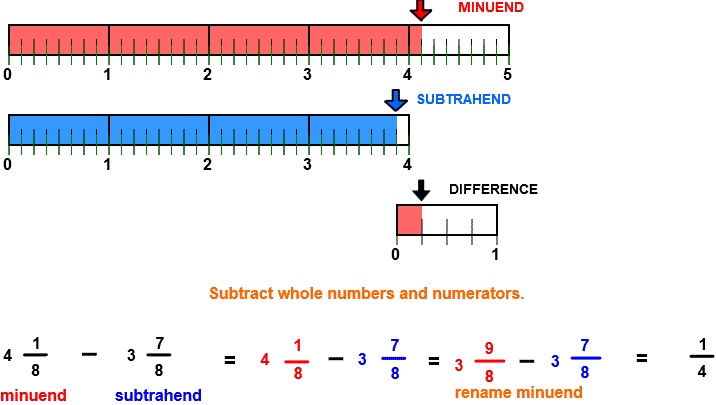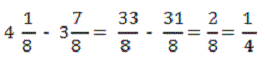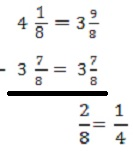# Subtract Like Fractions

## Subtract Like Fractions with Number Line Models

SUBTRACT LIKE FRACTIONS
CORRECT:
ATTEMPTS:
SCORE:
PERCENT
WHOLE
NUMERATOR
DENOMINATOR

# SUBTRACT LIKE FRACTIONS INSTRUCTIONS

Follow the directions in the dialog box after pressing the <START> button. The <EXPLAIN> button may be pressed after you enter the subtrahend to see how to do the example.

The parts of a subtraction example are the minuend, the subtrahend, and the difference.

When the program starts, you will be asked to identify the minuend and then the subtrahend. You will then be asked to find the difference.

The following image was made by Subtract Fractions Designer:Both minuend and subtrahend in this program have the same denominator. Fractions with the same denominators are like fractions.

The picture shows that the minuend is 4 18 units in length and the subtrahend is 3 78 units in length. The difference will be the distance from the end of the subtrahend to the end of the minuend for 28 or 14 units.

When the numerator of the subtrahend is larger than the numerator of the minuend you may rename the minuend to subtract. Some texts call the procedure "borrowing". To borrow, simply decrease the whole number 3 by 1. Then add 88 to 18 to get 98, giving the fraction 3 98.

Notice how 4 18 is renamed as 3 98 so that the numerators can be subtracted.

Another method would be to first write the minuend and subtrahend in fraction form. The example would look like this:Or you may prefer to work vertically. In this example the minuend 4 1/8 is written as 3 9/8.For more instruction on subtracting fractions go to How To Subtract Fractions.

Follow the directions in the dialog box after pressing the <START> button. The <EXPLAIN> button may be pressed after you enter the second addend to see how to do the example.

After you enter the difference you may press the <REPORT> button. The report will ask for your name but you may submit a code for your name. This report will give the same results as on the dialog box. The report may be printed or e-mailed.